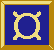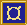# ¤ idSP, filterSP, et al

Stream processor equivalents of some common list processing functions

## Types

```idSP :: SP b b
filterSP :: (b -> Bool) -> SP b b
mapSP :: (t -> b) -> SP t b
concatMapSP :: (t -> [b]) -> SP t b
mapAccumlSP :: (t -> a -> (t, b)) -> t -> SP a b
concatMapAccumlSP :: (t -> a -> (t, [b])) -> t -> SP a b
concatSP :: SP [b] b
zipSP :: [a] -> SP b (a, b)
splitAtElemSP :: (a -> Bool) -> Cont (SP a b) [a]
chopSP :: ((b -> SP a b) -> SP a b) -> SP a b```

`type Cont c a = (a -> c) -> c`

## Description

These are some common stream processors. `idSP` is the identity stream processor, i.e., a short-circuit from its input to its output. `mapSP f` is a stream processor that outputs `f x` when it receives `x` on its input and thus is the equivalent of `map` for lists.

The other stream processors too are equivalent to the similarly named list functions.